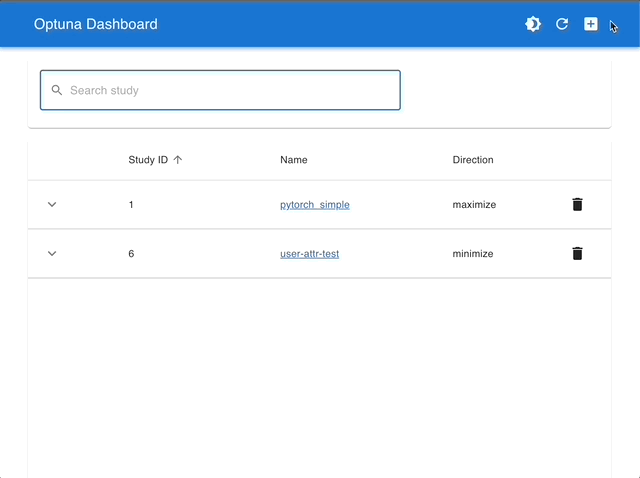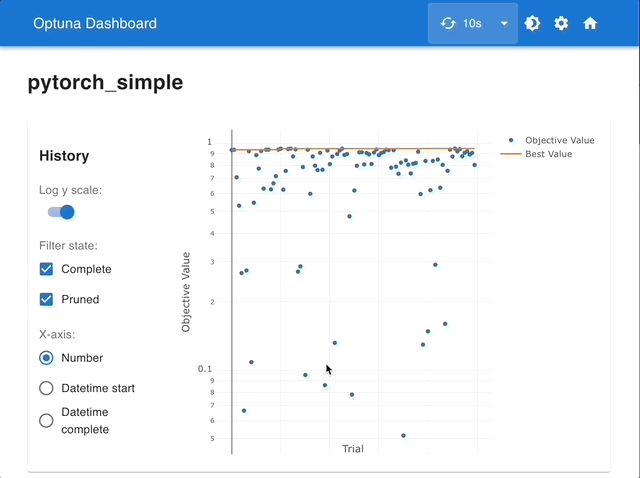# Quick Visualization for Hyperparameter Optimization Analysis

Optuna provides various visualization features in optuna.visualization to analyze optimization results visually.

This tutorial walks you through this module by visualizing the history of lightgbm model for breast cancer dataset.

For visualizing multi-objective optimization (i.e., the usage of optuna.visualization.plot_pareto_front()), please refer to the tutorial of Multi-objective Optimization with Optuna.

Note

By using Optuna Dashboard, you can also check the optimization history, hyperparameter importances, hyperparameter relationships, etc. in graphs and tables. Please make your study persistent using RDB backend and execute following commands to run Optuna Dashboard.

$pip install optuna-dashboard$ optuna-dashboard sqlite:///example-study.db


Please check out the GitHub repository for more details.

Manage Studies

Visualize with Interactive Graphsimport lightgbm as lgb
import numpy as np
import sklearn.datasets
import sklearn.metrics
from sklearn.model_selection import train_test_split

import optuna

# You can use Matplotlib instead of Plotly for visualization by simply replacing optuna.visualization with
# optuna.visualization.matplotlib in the following examples.
from optuna.visualization import plot_contour
from optuna.visualization import plot_edf
from optuna.visualization import plot_intermediate_values
from optuna.visualization import plot_optimization_history
from optuna.visualization import plot_parallel_coordinate
from optuna.visualization import plot_param_importances
from optuna.visualization import plot_rank
from optuna.visualization import plot_slice
from optuna.visualization import plot_timeline

SEED = 42

np.random.seed(SEED)


Define the objective function.

def objective(trial):
train_x, valid_x, train_y, valid_y = train_test_split(data, target, test_size=0.25)
dtrain = lgb.Dataset(train_x, label=train_y)
dvalid = lgb.Dataset(valid_x, label=valid_y)

param = {
"objective": "binary",
"metric": "auc",
"verbosity": -1,
"boosting_type": "gbdt",
"bagging_fraction": trial.suggest_float("bagging_fraction", 0.4, 1.0),
"bagging_freq": trial.suggest_int("bagging_freq", 1, 7),
"min_child_samples": trial.suggest_int("min_child_samples", 5, 100),
}

# Add a callback for pruning.
pruning_callback = optuna.integration.LightGBMPruningCallback(trial, "auc")
gbm = lgb.train(param, dtrain, valid_sets=[dvalid], callbacks=[pruning_callback])

preds = gbm.predict(valid_x)
pred_labels = np.rint(preds)
accuracy = sklearn.metrics.accuracy_score(valid_y, pred_labels)
return accuracy

study = optuna.create_study(
direction="maximize",
sampler=optuna.samplers.TPESampler(seed=SEED),
pruner=optuna.pruners.MedianPruner(n_warmup_steps=10),
)
study.optimize(objective, n_trials=100, timeout=600)


## Plot functions

Visualize the optimization history. See plot_optimization_history() for the details.

plot_optimization_history(study)


Visualize the learning curves of the trials. See plot_intermediate_values() for the details.

plot_intermediate_values(study)


Visualize high-dimensional parameter relationships. See plot_parallel_coordinate() for the details.

plot_parallel_coordinate(study)


Select parameters to visualize.

plot_parallel_coordinate(study, params=["bagging_freq", "bagging_fraction"])


Visualize hyperparameter relationships. See plot_contour() for the details.

plot_contour(study)


Select parameters to visualize.

plot_contour(study, params=["bagging_freq", "bagging_fraction"])


Visualize individual hyperparameters as slice plot. See plot_slice() for the details.

plot_slice(study)


Select parameters to visualize.

plot_slice(study, params=["bagging_freq", "bagging_fraction"])


Visualize parameter importances. See plot_param_importances() for the details.

plot_param_importances(study)


Learn which hyperparameters are affecting the trial duration with hyperparameter importance.

optuna.visualization.plot_param_importances(
study, target=lambda t: t.duration.total_seconds(), target_name="duration"
)


Visualize empirical distribution function. See plot_edf() for the details.

plot_edf(study)


Visualize parameter relations with scatter plots colored by objective values. See plot_rank() for the details.

plot_rank(study)

/home/docs/checkouts/readthedocs.org/user_builds/optuna/checkouts/v3.3.0/tutorial/10_key_features/005_visualization.py:147: ExperimentalWarning:

plot_rank is experimental (supported from v3.2.0). The interface can change in the future.


Visualize the optimization timeline of performed trials. See plot_timeline() for the details.

plot_timeline(study)

/home/docs/checkouts/readthedocs.org/user_builds/optuna/checkouts/v3.3.0/tutorial/10_key_features/005_visualization.py:151: ExperimentalWarning:

plot_timeline is experimental (supported from v3.2.0). The interface can change in the future.


Total running time of the script: ( 0 minutes 5.457 seconds)

Gallery generated by Sphinx-Gallery